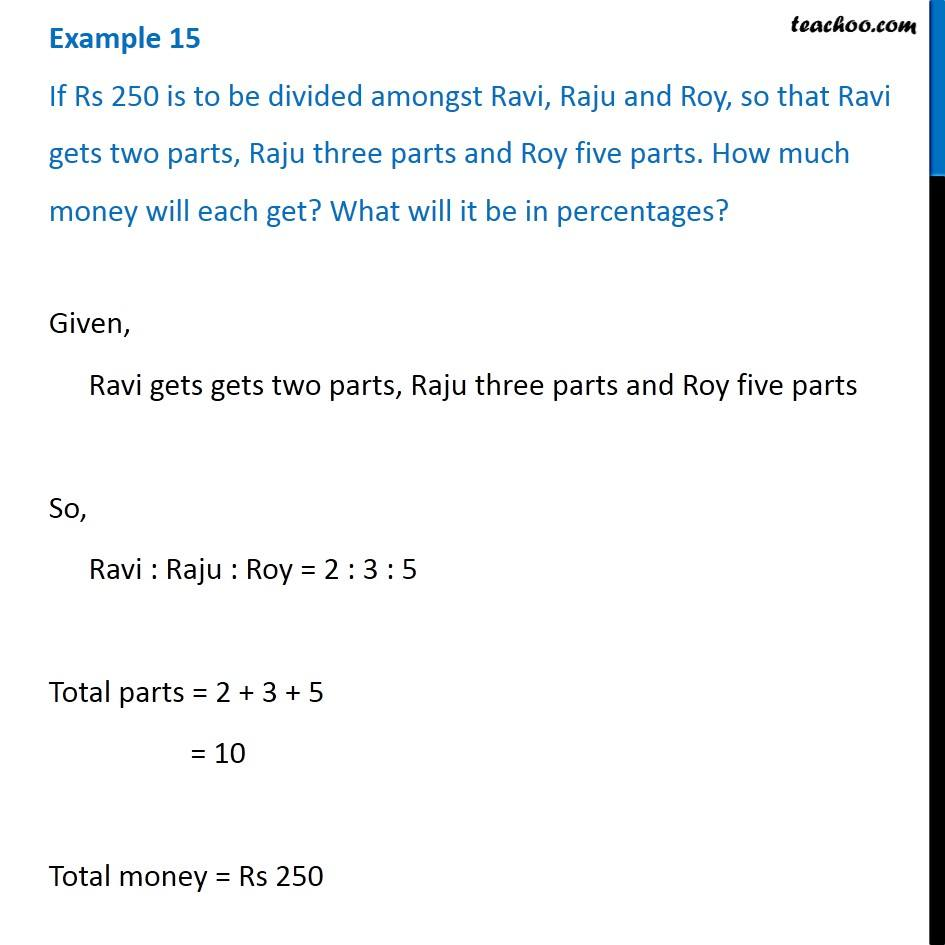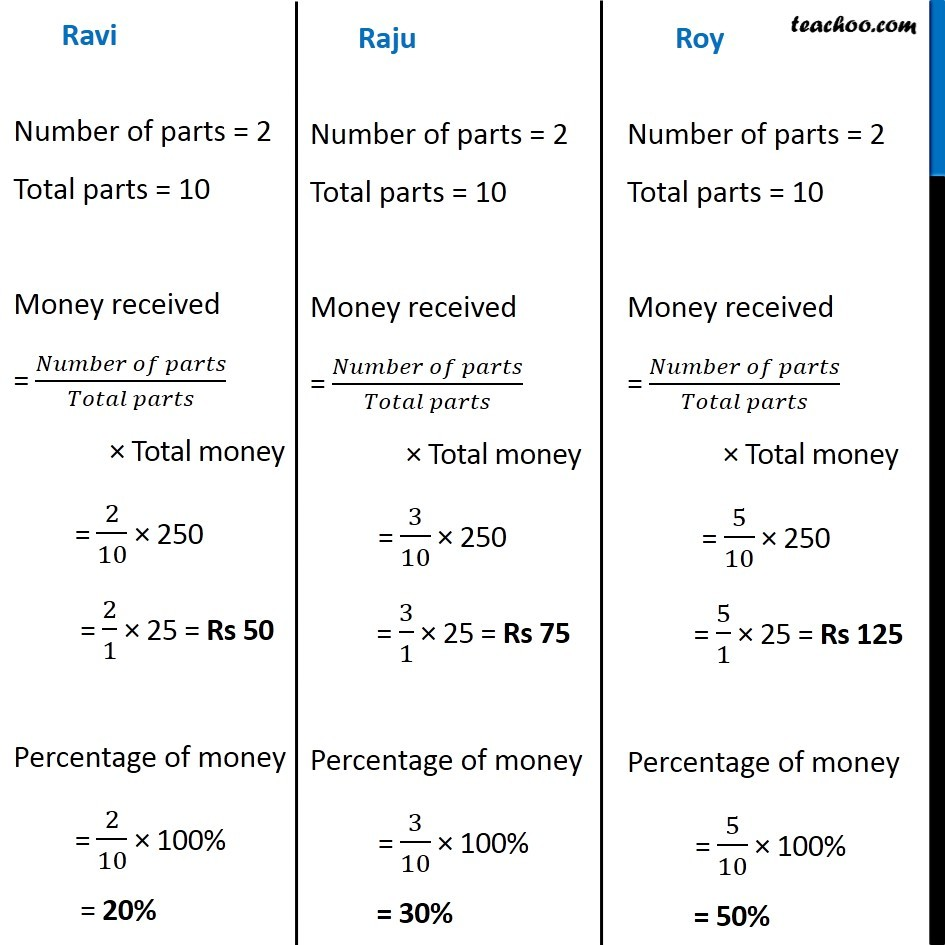Ratios to percentage

Chapter 7 Class 7 Comparing Quantities
Concept wiseLearn in your speed, with individual attention - Teachoo Maths 1-on-1 Class

### Transcript

Example 9 If Rs 250 is to be divided amongst Ravi, Raju and Roy, so that Ravi gets two parts, Raju three parts and Roy five parts. How much money will each get? What will it be in percentages? Given, Ravi gets gets two parts, Raju three parts and Roy five parts So, Ravi : Raju : Roy = 2 : 3 : 5 Total parts = 2 + 3 + 5 = 10 Total money = Rs 250 Ravi Number of parts = 2 Total parts = 10 Money received = (𝑁𝑢𝑚𝑏𝑒𝑟 𝑜𝑓 𝑝𝑎𝑟𝑡𝑠)/(𝑇𝑜𝑡𝑎𝑙 𝑝𝑎𝑟𝑡𝑠) × Total money = 2/10 × 250 = 2/1 × 25 = Rs 50 Percentage of money = 2/10 × 100% = 20% Raju Number of parts = 2 Total parts = 10 Money received = (𝑁𝑢𝑚𝑏𝑒𝑟 𝑜𝑓 𝑝𝑎𝑟𝑡𝑠)/(𝑇𝑜𝑡𝑎𝑙 𝑝𝑎𝑟𝑡𝑠) × Total money = 3/10 × 250 = 3/1 × 25 = Rs 75 Percentage of money = 3/10 × 100% = 30% Roy Number of parts = 2 Total parts = 10 Money received = (𝑁𝑢𝑚𝑏𝑒𝑟 𝑜𝑓 𝑝𝑎𝑟𝑡𝑠)/(𝑇𝑜𝑡𝑎𝑙 𝑝𝑎𝑟𝑡𝑠) × Total money = 5/10 × 250 = 5/1 × 25 = Rs 125 Percentage of money = 5/10 × 100% = 50%